Courses

# Second Law Of Thermodynamics MCQ Level – 2 (Part - 2)

## 10 Questions MCQ Test Topic wise Tests for IIT JAM Physics | Second Law Of Thermodynamics MCQ Level – 2 (Part - 2)

Description
This mock test of Second Law Of Thermodynamics MCQ Level – 2 (Part - 2) for IIT JAM helps you for every IIT JAM entrance exam. This contains 10 Multiple Choice Questions for IIT JAM Second Law Of Thermodynamics MCQ Level – 2 (Part - 2) (mcq) to study with solutions a complete question bank. The solved questions answers in this Second Law Of Thermodynamics MCQ Level – 2 (Part - 2) quiz give you a good mix of easy questions and tough questions. IIT JAM students definitely take this Second Law Of Thermodynamics MCQ Level – 2 (Part - 2) exercise for a better result in the exam. You can find other Second Law Of Thermodynamics MCQ Level – 2 (Part - 2) extra questions, long questions & short questions for IIT JAM on EduRev as well by searching above.
QUESTION: 1

### Consider a system of N atoms of an ideal gas of type A at temperature T and volume V. It is kept in diffusive contact with another system of N atoms of another ideal gas of type B at the same temperature T and volume V. Once the combined system reaches equilibrium. Select one:

Solution:

The correct answer is: entropy of final system is equal to the sum of individual entropies

QUESTION: 2

Solution:
QUESTION: 3

### For the thermodynamics process shown in the figure, corresponding T-S diagram is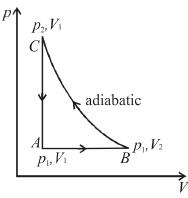Select one:

Solution:
QUESTION: 4

The T–S diagram of two cycles for the operation of an engine are shown in figure below.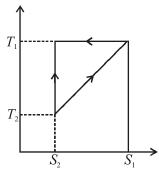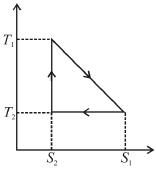The numerical values of the parameters T1 , T2 , S1 and S2 in the two figures are the same, then which cycle has greater efficiency?
Select one:

Solution:

Efficiency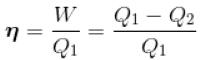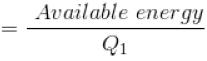For curve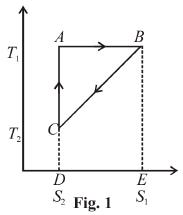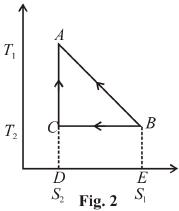Available energy per cycle is
Q1 – Q2 = Area of curve (ABC)
Q1 = Total area of curve (ABED)
⇒ Area of curve is same for both figures, but total area is more for Fig. 1.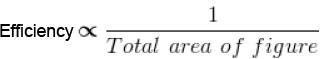Hence, second figure has greater efficiency.

QUESTION: 5

An electric current of 3A flows through a resistance of 10 ohm. It is being cooled by running water and is kept at temperature 300K, change in entropy per second of the resistance :
Select one:

Solution:

There is no change in the entropy of the resistance because there is no change in the state of wire.
The correct answer is: No change

QUESTION: 6

Out of three Carnot engines, operating between reservoir temperature of (a) 400 and 500 K (b) 600 and 800 K (c) 400 and 600 K, which has the greater thermal efficiency?

Solution:
QUESTION: 7

A reversible engine cyclic is shown in the following T–S diagram. The efficiency of the engine is :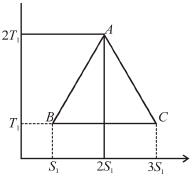Select one:

Solution:

In given figure, available energy per cycle is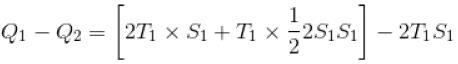= T1S1
and    Q1 = 3T1S1
efficiency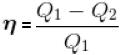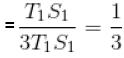QUESTION: 8

An engine absorbs heat at temperature of 1000 K and rejects heat at 600 K. If the engine operates at maximum possible efficiency, the amount of work performed by the engine for 200 J heat input is
Select one:

Solution:
QUESTION: 9

An amount of heat Q is transferred from a heat reservoir at temperature TA to another heat reservoir at temperature TB. What is the change in the entropy Δs of the combined system?
Select one:

Solution:

Conventionally, heat given to the system is taken to be positive and heat extracted from the system is taken to be negative.
When heat is changed in the system entropy changed by an amount,
∴ For A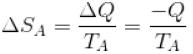Similarly for B,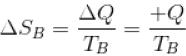ΔS = ΔSA + ΔSB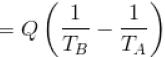The correct answer is: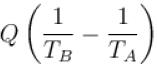QUESTION: 10

The difference in entropy between a state of volume Vi and a state of volume Vf (temperature and number of molecules remaining constant) is equal to :
Select one:

Solution: